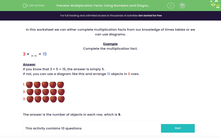# Multiplication Facts: Using Numbers and Diagrams

In this worksheet, students complete multiplication facts either from their knowledge of times tables or using objects.Key stage:  KS 2

Curriculum topic:   Number: Multiplication and Division

Curriculum subtopic:   Solve Multiplication/Division Problems

Difficulty level:#### Worksheet Overview

In this worksheet we can either complete multiplication facts from our knowledge of times tables or we can use diagrams.

Example

Complete the multiplication fact.

3 × __ = 15

If you know that 3 × 5 = 15, the answer is simply 5.

If not, you can use a diagram like this and arrange 15 objects in 3 rows.

1.2.3.The answer is the number of objects in each row, which is 5.

### What is EdPlace?

We're your National Curriculum aligned online education content provider helping each child succeed in English, maths and science from year 1 to GCSE. With an EdPlace account you’ll be able to track and measure progress, helping each child achieve their best. We build confidence and attainment by personalising each child’s learning at a level that suits them.

Get started